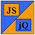# JavaScript & jQuery Tutorials

## Project

In this section of the website we utilise what we have learnt from The JavaScript and jQuery tutorials to create a Project. The Project consists of a proposal for an interactive quiz followed by lessons in which we create all the other components starting with the HTML and CSS and then adding The JavaScript and jQuery.

## Lesson  1: Interactive Quiz Proposal

In this lesson we look at an idea for an interactive quiz for The JavaScript and jQuery Project.

## Lesson  2: Quiz Layout

In this lesson we will create the skeleton HTML and CSS for our interactive quiz by looking at the action points raised in the last lesson and extracting the information required to do this.

## Lesson  3: Refining The Layout

In this lesson we will refine the skeleton HTML and CSS we created in the last lesson by looking at some more of the action points concerned with structure and presentation.

In this lesson we will create navigation for our interactive quiz using JavaScript and jQuery so we can traverse backwards and forwards through the quiz questions.

## Lesson  5: Quiz Feedback

In this lesson we finish off our action points by giving the user feedback on how they did in the quiz.

## Lesson  6: Enhancing The Quiz

In this lesson we enhance the interactive quiz to dynamically calculate the number of questions and give better feedback on quiz completion.

## Lesson  7: Quiz Summary

In this lesson we summarize the HTML, CSS, JavaScript and jQuery used for our Project.

## What's Next

In Lesson 1 we look at an idea for an interactive quiz for The JavaScript and jQuery Project.

Interactive Quiz Proposal  >>